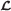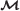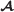# Variance of uncertain random variables

## Abstract

Uncertain random variable is developed to describe the phenomenon which mixes uncertainty with randomness. The variance of uncertain random variable may provide a degree of the spread of the distribution around its expected value. This paper presents a formula to obtain the variance of uncertain random variable.

## 1 Introduction

Prior to today, probability theory and fuzzy set theory are two common mathematical tools to model indeterminacy phenomena and have been widely applied in information theory, engineering, management science, and so on. We know a fundamental premise of applying probability theory is that the estimated probability is closed enough to the real frequency. However, such as ‘strength of bridge’, ‘about one million tons’, ‘tall’, and ‘most’, due to lack of observed data and the complexity of environment, when making decisions, people have to consult with domain experts. In this case, information and knowledge cannot be described well by random variables. For fuzzy set theory, it was still challenged by many scholars after it was founded. Liu  presented several paradoxes to show that fuzzy variable and fuzzy set are not suitable for modeling uncertain quantities and unsharp concepts, respectively.

In order to deal with non-random phenomena, an uncertainty theory was founded by Liu  and refined by Liu  and then became a branch of mathematics for modeling belief degrees. One the hand, Liu presented some basic and important theoretical works of uncertainty theory . On the other hand, as an application of uncertainty theory, Liu  proposed a spectrum of uncertain programming which is a type of mathematical programming involving uncertain variables and applied uncertain programming to system reliability design, facility location problem, vehicle routing problem, project scheduling problem, finance, and so on. In order to well interpret expert’s experimental data by uncertainty theory, Liu first proposed uncertain statistics, and Wang et al. build several uncertain statistical methods such as the method of moments , uncertain Delphi method , and uncertain hypothesis testing for expert’s empirical data  and discussed uncertain variance of sample and its application . Nowadays, uncertainty theory was well developed on both theory section and practice section. For exploring the recent developments of uncertainty theory, the readers may consult the book .

Inspired by Kwakernaak [9, 10], Puri and Ralescu , Kruse and Meyer , and Liu and Liu [13, 14], uncertain random variable was first defined by Liu  to describe complex systems in which uncertainty and randomness frequently appear together. Otherwise, in order to model uncertain random phenomena well, Liu  also introduce the concepts of uncertain random arithmetic, chance measure, chance distribution, expected value, variance, and so on. As everyone knows, the variance of uncertain random variable will provide a degree of the spread of the distribution around its expected value. Although the concept of variance for uncertain random variable has been defined by expected value, we still do not know how to obtain the variance from chance distribution. This paper will build the formula for the convenience of obtaining the variance from chance distribution. The remainder of this paper is organized as follows. The next section is intended to introduce some concepts in uncertainty theory as they are needed. A formula for obtaining the variance of uncertain random variable and some examples are proposed in Section 3. Finally, a conclusion is drawn in Section 4.

## 2 Preliminary

Uncertainty theory was founded by Liu  in 2007 and refined by Liu  in 2010 and became a branch of mathematics based on normality, duality, subadditivity, and product axioms. For exploring the recent developments of uncertainty theory, the readers can consult the book by Liu . Based on uncertainty theory and probability theory, chance theory was pioneered by Liu  in order to model the uncertain random compound systems. In this section, some useful concepts are introduced. Let $\left(\Gamma ,ℒ,ℳ\right)$ be an uncertainty space, where Γ is a non-empty set,is a σ-algebra defined on Γ, andis an uncertain measure, and let $\left(\Omega ,A,\mathit{\text{Pr}}\right)$ be a probability space, where Ω is a sample space,is a σ-algebra defined on Ω, and Pr is a probability measure.

### Definition2.1

An uncertain random variable is a function ξ from a chance space $\left(\Gamma ,ℒ,ℳ\right)×\left(\Omega ,A,\mathit{\text{Pr}}\right)$ to the set of real numbers such that {ξB} is an event in $ℒ×A$ for any Borel set B.

### Definition2.2.

Let ξ be an uncertain random variable on the chance space $\left(\Gamma ,ℒ,ℳ\right)×\left(\Omega ,A,\mathit{\text{Pr}}\right)$, and let B be a Borel set. Then, {ξB} is an uncertain random event with chance measure 

$\mathit{\text{Ch}}\left\{\xi \in B\right\}={\int }_{0}^{1}\mathit{\text{Pr}}\left\{\omega \in \Omega \mid ℳ\left\{\gamma \in \Gamma \mid \xi \left(\gamma ,\omega \right)\in B\right\}\ge x\right\}\mathrm{dx.}$
(2.1)

Note that the chance measure is in fact the expected value of the random variable $ℳ\left\{\xi \left(·\right)\in B\right\}$, i.e.,

$\mathit{\text{Ch}}\left\{\xi \in B\right\}=E\left[\phantom{\rule{0.3em}{0ex}}ℳ\left\{\xi \left(·\right)\in B\right\}\right],$
(2.2)

where E denotes the expected value operator for random variable under probability measure Pr.

### Theorem 2.1

Let ξ be an uncertain random variable. Then, the chance measure C h{ξB} has normality, duality, and monotonicity properties, i.e., 

1. (i)

C h{ξ}=1,

2. (ii)

C h{ξB}+C h{ξB c}=1, for any Borel set B,

3. (iii)

C h{ξB 1}≤C h{ξB 2}, for any Borel sets B 1B 2.

### Definition2.3.

Let ξ be an uncertain random variable. Then, its chance distribution of ξ is defined by 

$\Phi \left(x\right)=\mathit{\text{Ch}}\left\{\xi \le x\right\},\phantom{\rule{1em}{0ex}}\text{for any}\phantom{\rule{1em}{0ex}}x\in \Re \text{.}$

An operational law of uncertain random variables was given by Liu  as follows.

### Theorem2.2.

Let η1, η2,, η m be independent random variables with probability distributions Ψ1, Ψ2,, Ψ m , and let τ1, τ2,, τ n be independent uncertain variables with uncertainty distributions Υ1, Υ2,, Υ n , respectively. Then, the uncertain random variable ξ=f(η1, η2,, η m , τ1, τ2,, τ n ) has a chance distribution 

$\Phi \left(x\right)={\int }_{{\Re }^{m}}F\left(x;{y}_{1},{y}_{2},\cdots \phantom{\rule{0.3em}{0ex}},{y}_{m}\right){d\Psi }_{1}\left(\phantom{\rule{0.3em}{0ex}}{y}_{1}\right){d\Psi }_{2}\left(\phantom{\rule{0.3em}{0ex}}{y}_{2}\right){\cdots d\Psi }_{m}\left(\phantom{\rule{0.3em}{0ex}}{y}_{m}\right),$
(2.3)

where F(x;y1, y2,, y m ) is determined by its inverse function

${F}^{-1}\left({\alpha ;y}_{1},{y}_{2},\cdots \phantom{\rule{0.3em}{0ex}},{y}_{m}\right)=f\left(\phantom{\rule{0.3em}{0ex}}{y}_{1},{y}_{2},\cdots \phantom{\rule{0.3em}{0ex}},{y}_{m},{Υ}_{1}^{-1}\left(\alpha \right),{Υ}_{2}^{-1}\left(\alpha \right),\cdots \phantom{\rule{0.3em}{0ex}},{Υ}_{n}^{-1}\left(\alpha \right)\right)$
(2.4)

provided that f(η1, η2,, η m , τ1, τ2,, τ n ) is a strictly increasing function with respect to τ1, τ2,, τ n .

Note that if η1, η2,, η m are non-independent random variables with joint distribution Ψ(y1, y2,, y m ), Equation 2.3 can be modified as below,

$\Phi \left(x\right)={\int }_{{\Re }^{m}}F\left(x;{y}_{1},{y}_{2},\cdots \phantom{\rule{0.3em}{0ex}},{y}_{m}\right)\mathrm{d\Psi }\left({y}_{1},{y}_{2},\cdots \phantom{\rule{0.3em}{0ex}},{y}_{m}\right).$
(2.5)

### Definition2.4.

Let ξ be an uncertain random variable. Then its expected value is defined by 

$E\left[\phantom{\rule{0.3em}{0ex}}\xi \right]={\int }_{0}^{+\infty }\mathit{\text{Ch}}\left\{\xi \ge r\right\}\mathit{\text{dr}}-{\int }_{-\infty }^{0}\mathit{\text{Ch}}\left\{\xi \le r\right\}\mathit{\text{dr}}$
(2.6)

provided that at least one of the two integrals is finite.

### Theorem2.3.

Let η1, η2,, η m be independent random variables with probability distributions Ψ1, Ψ2,, Ψ m , respectively, and let τ1, τ2,, τ n be uncertain variables, then the uncertain random variable 

$\begin{array}{l}\xi =f\left({\eta }_{1},\cdots \phantom{\rule{0.3em}{0ex}},{\eta }_{m},{\tau }_{1},\cdots \phantom{\rule{0.3em}{0ex}},{\tau }_{n}\right)\end{array}$

has an expected value

$E\left[\phantom{\rule{0.3em}{0ex}}\xi \right]={\int }_{{\Re }^{m}}E\left[f\left(\phantom{\rule{0.3em}{0ex}}{y}_{1},\cdots \phantom{\rule{0.3em}{0ex}},{y}_{m},{\tau }_{1},\cdots \phantom{\rule{0.3em}{0ex}},{\tau }_{n}\right)\right]{d\Psi }_{1}\left(\phantom{\rule{0.3em}{0ex}}{y}_{1}\right){\cdots d\Psi }_{m}\left({y}_{m}\right)$

where E[f(y1,, y m , τ1,, τ n )] is the expected value of the uncertain variable f(η1,, η m , τ1,, τ n ) for any real numbers y1,, y m .

As a special case, Liu  proved the following theorem.

### Theorem2.4.

Let η be a random variable and let τ be an uncertain variable . Then

$E\left[\phantom{\rule{0.3em}{0ex}}\eta +\tau \right]=E\left[\phantom{\rule{0.3em}{0ex}}\eta \right]+E\left[\phantom{\rule{0.3em}{0ex}}\tau \right]$
(2.7)

and

$E\left[\phantom{\rule{0.3em}{0ex}}\mathrm{\eta \tau }\right]=E\left[\phantom{\rule{0.3em}{0ex}}\eta \right]E\left[\phantom{\rule{0.3em}{0ex}}\tau \right].$
(2.8)

## 3 Variance of uncertain random variables

A formal definition of variance for uncertain random variable was presented by Liu  as follows.

### Definition3.1.

Let ξ be an uncertain random variable with expected value e. Then, its variance is defined by 

$V\left[\phantom{\rule{0.3em}{0ex}}\xi \right]=E\left[\phantom{\rule{0.3em}{0ex}}{\left(\xi -e\right)}^{2}\right].$
(3.9)

The variance of uncertain random variable provides a degree of spread of the distribution around its expected value. The following theorem presents a formula to obtain the variance for a class of uncertain random variable.

### Theorem3.1.

Let η1, η2,, η m be independent random variables with probability distributions Ψ1, Ψ2,, Ψ m , respectively, and let τ1, τ2,, τ n be uncertain variables; then, the uncertain random variable

$\xi =f\left({\eta }_{1},\cdots \phantom{\rule{0.3em}{0ex}},{\eta }_{m},{\tau }_{1},\cdots \phantom{\rule{0.3em}{0ex}},{\tau }_{n}\right)$

has variance

$\phantom{\rule{-20.0pt}{0ex}}V\left[\phantom{\rule{0.3em}{0ex}}\xi \right]=2{\int }_{{R}^{m}}{\int }_{0}^{\infty }x\left[1-F\left(e+x;{y}_{1},\cdots \phantom{\rule{0.3em}{0ex}},{y}_{m}\right)+F\left(e-x;{y}_{1},\cdots \phantom{\rule{0.3em}{0ex}},{y}_{m}\right)\right]{\mathit{\text{dxd}}\Psi }_{1}\left({y}_{1}\right){\cdots d\Psi }_{m}\left({y}_{m}\right)$
(3.10)

where

$F\left(x;{y}_{1},\cdots \phantom{\rule{0.3em}{0ex}},{y}_{m}\right)=ℳ\left\{\phantom{\rule{0.3em}{0ex}}f\left(\phantom{\rule{0.3em}{0ex}}{y}_{1},\cdots \phantom{\rule{0.3em}{0ex}},{y}_{m},{\tau }_{1},\cdots \phantom{\rule{0.3em}{0ex}},{\tau }_{n}\right)\le x\right\}$

and

$e={\int }_{{\Re }^{m}}E\left[f\left({y}_{1},\cdots \phantom{\rule{0.3em}{0ex}},{y}_{m},{\tau }_{1},\cdots \phantom{\rule{0.3em}{0ex}},{\tau }_{n}\right)\right]{d\Psi }_{1}\left({y}_{1}\right){\cdots d\Psi }_{m}\left({y}_{m}\right)$

are the uncertainty distribution and expected value of uncertain random variable ξ, respectively.

### Proof.

Suppose that the uncertain random variable ξ=f(η1,, η m , τ1,, τ n ) has the expected value e, by the definitions of variance and chance measure, we have

$\begin{array}{ll}V\left[\phantom{\rule{0.3em}{0ex}}\xi \right]& ={\int }_{0}^{+\infty }\mathit{\text{Ch}}\left\{{\left(\xi -e\right)}^{2}\ge x\right\}\mathit{\text{dx}}\\ {={\int }_{0}^{+\infty }{E}_{\mathit{\text{Pr}}}\left[\phantom{\rule{0.3em}{0ex}}ℳ\left\{\xi -e\right)}^{2}\ge x\right\}\right]\mathit{\text{dx}}\\ ={\int }_{0}^{+\infty }{E}_{\mathit{\text{Pr}}}\left[\phantom{\rule{0.3em}{0ex}}ℳ\left\{{\left(\phantom{\rule{0.3em}{0ex}}f\left({\eta }_{1},\cdots \phantom{\rule{0.3em}{0ex}},{\eta }_{m},{\tau }_{1},\cdots \phantom{\rule{0.3em}{0ex}},{\tau }_{n}\right)-e\right)}^{2}\ge x\right\}\right]\mathit{\text{dx}}\\ ={\int }_{0}^{+\infty }{\int }_{{R}^{m}}ℳ\left\{{\left(\phantom{\rule{0.3em}{0ex}}f\left(\phantom{\rule{0.3em}{0ex}}{y}_{1},\cdots \phantom{\rule{0.3em}{0ex}},{y}_{m};{\tau }_{1},\cdots \phantom{\rule{0.3em}{0ex}},{\tau }_{n}\right)-e\right)}^{2}{\ge x\right\}d\Psi }_{1}\left(\phantom{\rule{0.3em}{0ex}}{y}_{1}\right)\cdots d{\Psi }_{m}\left(\phantom{\rule{0.3em}{0ex}}{y}_{m}\right)\mathit{\text{dx}}\\ ={\int }_{{R}^{m}}{\int }_{0}^{+\infty }ℳ\left\{{\left(\phantom{\rule{0.3em}{0ex}}f\left(\phantom{\rule{0.3em}{0ex}}{y}_{1},\cdots \phantom{\rule{0.3em}{0ex}},{y}_{m};{\tau }_{1},\cdots \phantom{\rule{0.3em}{0ex}},{\tau }_{n}\right)-e\right)}^{2}{\ge x\right\}\mathit{\text{dxd}}\Psi }_{1}\left(\phantom{\rule{0.3em}{0ex}}{y}_{1}\right)\cdots d{\Psi }_{m}\left(\phantom{\rule{0.3em}{0ex}}{y}_{m}\right).\end{array}$
(3.11)

For the above equation, we have

$\begin{array}{r}{\int }_{0}^{+\infty }ℳ\left\{{\left(f\left({y}_{1},\cdots \phantom{\rule{0.3em}{0ex}},{y}_{m};{\tau }_{1},\cdots \phantom{\rule{0.3em}{0ex}},{\tau }_{n}\right)-e\right)}^{2}\ge x\right\}\mathit{\text{dx}}\\ =& {\int }_{0}^{+\infty }ℳ\left\{f\left({y}_{1},\cdots \phantom{\rule{0.3em}{0ex}},{y}_{m};{\tau }_{1},\cdots \phantom{\rule{0.3em}{0ex}},{\tau }_{n}\right)\ge e+\sqrt{x}\cup f\left({y}_{1},\cdots \phantom{\rule{0.3em}{0ex}},{y}_{m};{\tau }_{1},\cdots \phantom{\rule{0.3em}{0ex}},{\tau }_{n}\right)\le e-\sqrt{x}\right\}\mathit{\text{dx}}\\ \le & {\int }_{0}^{+\infty }ℳ\left\{f\left({y}_{1},\cdots \phantom{\rule{0.3em}{0ex}},{y}_{m};{\tau }_{1},\cdots \phantom{\rule{0.3em}{0ex}},{\tau }_{n}\right)\ge e+\sqrt{x}\right\}\mathit{\text{dx}}\\ \phantom{\rule{1em}{0ex}}+& {\int }_{0}^{+\infty }ℳ\left\{f\left({y}_{1},\cdots \phantom{\rule{0.3em}{0ex}},{y}_{m};{\tau }_{1},\cdots \phantom{\rule{0.3em}{0ex}},{\tau }_{n}\right)\le e-\sqrt{x}\right\}\mathit{\text{dx}}\\ =& {\int }_{0}^{+\infty }\left[\phantom{\rule{0.3em}{0ex}}1-F\left(e+\sqrt{x};{y}_{1},\cdots \phantom{\rule{0.3em}{0ex}},{y}_{m}\right)+F\left(e-\sqrt{x};{y}_{1},\cdots \phantom{\rule{0.3em}{0ex}},{y}_{m}\right)\right]\mathit{\text{dx}}\\ =& 2{\int }_{0}^{+\infty }x\left[\phantom{\rule{0.3em}{0ex}}1-F\left(e+x;{y}_{1},\cdots \phantom{\rule{0.3em}{0ex}},{y}_{m}\right)+F\left(e-x;{y}_{1},\cdots \phantom{\rule{0.3em}{0ex}},{y}_{m}\right)\right]\mathit{\text{dx}},\end{array}$
(3.12)

where F(x;y1,, y m ) is the uncertainty distribution of uncertain variable f(y1,, y m ;τ1,, τ n ) for any real numbers y1, y2,, y m .

By Equations 3.11 and 3.12, this theorem is proved.

### Example1.

Let η be a random variable with probability distribution Ψ, and let τ be an uncertain variable with uncertainty distribution Υ. Then, the sum

$\xi =\eta +\tau$

has the variance

$V\left[\phantom{\rule{0.3em}{0ex}}\xi \right]=2{\int }_{-\infty }^{+\infty }{\int }_{0}^{+\infty }x\left[\phantom{\rule{0.3em}{0ex}}1-Υ\left(e+x-y\right)+Υ\left(e-x-y\right)\right]\mathrm{dxd\Psi }\left(y\right),$

where e is the expected value of uncertain random variable ξ and the above integral is finite.

Especially, let η be a random variable having probability density function

$\varphi \left(x\right)=\left\{\begin{array}{cc}\frac{1}{d\phantom{\rule{0.3em}{0ex}}-\phantom{\rule{0.3em}{0ex}}c},& \phantom{\rule{0.3em}{0ex}}\phantom{\rule{0.3em}{0ex}}\text{if}\phantom{\rule{0.3em}{0ex}}\phantom{\rule{0.3em}{0ex}}c\le x\le d\\ 0,& \phantom{\rule{0.3em}{0ex}}\phantom{\rule{0.3em}{0ex}}\text{otherwise},\end{array}\right\$
(3.13)

and let τ be an linear uncertain variable with uncertainty distribution

$Υ\left(x\right)=\left\{\begin{array}{cc}0,& \phantom{\rule{0.3em}{0ex}}\phantom{\rule{0.3em}{0ex}}\text{if}\phantom{\rule{0.3em}{0ex}}\phantom{\rule{0.3em}{0ex}}x
(3.14)

By Theorem 2.3, the su

$\xi =\eta +\tau$

has the variance

$V\left[\phantom{\rule{0.3em}{0ex}}\xi \right]=\frac{{\left(d-c\right)}^{2}}{12}+\frac{{\left(b-a\right)}^{2}}{12}=V\left[\phantom{\rule{0.3em}{0ex}}\eta \right]+V\left[\phantom{\rule{0.3em}{0ex}}\tau \right].$

### Example2.

Let η be a random variable with probability distribution Ψ, and let τ be an uncertain variable with uncertainty distribution Υ. Then, the product

$\xi =\mathrm{\eta \tau }$

has the variance

$V\left[\phantom{\rule{0.3em}{0ex}}\xi \right]=2{\int }_{-\infty }^{+\infty }{\int }_{0}^{+\infty }x\left[1-Υ\left(\frac{e+x}{y}\right)+Υ\left(\frac{e-x}{y}\right)\right]\mathrm{dxd\Psi }\left(y\right),$

where e is the expected value of uncertain random variable ξ and the above integral is finite.

Especially, let η be a random variable having probability density function

$\varphi \left(x\right)=\left\{\begin{array}{cc}\frac{1}{d\phantom{\rule{0.3em}{0ex}}-\phantom{\rule{0.3em}{0ex}}c},& \phantom{\rule{0.3em}{0ex}}\phantom{\rule{0.3em}{0ex}}\text{if}\phantom{\rule{0.3em}{0ex}}\phantom{\rule{0.3em}{0ex}}0\le c\le x\le d\\ 0,& \phantom{\rule{0.3em}{0ex}}\phantom{\rule{0.3em}{0ex}}\text{otherwise}\end{array}\right\$
(3.15)

and let τ be an linear uncertain variable with uncertainty distribution

$Υ\left(x\right)=\left\{\begin{array}{cc}0,& \phantom{\rule{0.3em}{0ex}}\phantom{\rule{0.3em}{0ex}}\text{if}\phantom{\rule{0.3em}{0ex}}\phantom{\rule{0.3em}{0ex}}x
(3.16)

Then, the product

$\xi =\mathrm{\eta \tau }$

has the variance

$V\left[\phantom{\rule{0.3em}{0ex}}\xi \right]=-\frac{1}{16}{\left(a+b\right)}^{2}{\left(c+d\right)}^{2}+\frac{1}{9}\left({a}^{2}+{b}^{2}+\mathit{\text{ab}}\right)\left({c}^{2}+{d}^{2}+\mathit{\text{cd}}\right).$

### Remarks3.1

The variance of uncertain random variable ξ provides a degree of spread of the distribution around its expected value e. If we only know its chance distribution Φ, then the variance

$\begin{array}{rcl}V\left[\phantom{\rule{0.3em}{0ex}}\xi \right]& =& {\int }_{0}^{+\infty }\mathit{\text{Ch}}\left\{{\left(\xi -e\right)}^{2}\ge x\right\}\mathit{\text{dx}}\\ =& {\int }_{0}^{+\infty }\mathit{\text{Ch}}\left\{\left(\xi \ge e+\sqrt{x}\right)\cup \left(\xi \le e-\sqrt{x}\right)\right\}\mathit{\text{dx}}\\ \le & {\int }_{0}^{+\infty }\left(\mathit{\text{Ch}}\left\{\xi \ge e+\sqrt{x}\right\}+\mathit{\text{Ch}}\left\{\xi \le e-\sqrt{x}\right\}\right)\mathit{\text{dx}}\\ =& {\int }_{0}^{+\infty }\left(1-\Phi \left(e+\sqrt{x}\right)+\Phi \left(e-\sqrt{x}\right)\right)\mathrm{dx.}\end{array}$

Then, we have the following stipulation

$V\left[\phantom{\rule{0.3em}{0ex}}\xi \right]={\int }_{0}^{+\infty }\left(1-\Phi \left(e+\sqrt{x}\right)+\Phi \left(e-\sqrt{x}\right)\right)\mathrm{dx.}$

## 4 Conclusions

Uncertain random variable and chance theory were introduced to model the uncertain random compound systems. As the same as random variable and uncertain variable, expected value is the average of uncertain random variable in the sense of chance measure and the variance of uncertain random variable provides a degree of spread of the distribution around its expected value. In this paper, a series of formulas were built to obtain the variance of uncertain random variable.

## References

1. 1. Liu B: Uncertainty theory, 4th ed. 2014 http://orsc.edu.cn/liu/ut.pdf

2. Liu B: Uncertainty Theory. Berlin: Springer-Verlag; 2007.

3. Liu B: Uncertainty Theory: A Branch of Mathematics for Modeling Human Uncertainty. Berlin: Springer-Verlag; 2010.

4. Liu B: Theory and Practice of Uncertain Programming. Berlin: Springer-Verlag; 2009.

5. Wang X, Peng Z: Method of moments for estimating uncertainty distribution. J. Uncertainty Anal. Applications 2014, 2(5):1–12.

6. Wang X, Gao Z, Guo H: Delphi method for estimating uncertainty distributions. Inform. An Int. Interdiscip. J 2012, 15(2):449–460.

7. Wang X, Gao Z, Guo H: Uncertain hypothesis testing for two experts empirical data. Math. Comput. Model 2012, 55: 1478–1482. 10.1016/j.mcm.2011.10.039

8. Wang X, Guo H: Uncertain variance of sample and its application. Inform.: An Int. Interdiscip. J 2011, 14(1):79–88.

9. Kwakernaak H: Fuzzy random variables-1: definitions and theorems. Inform. Sci 1978, 15: 1–29. 10.1016/0020-0255(78)90019-1

10. Kwakernaak H: Fuzzy random variables-2: algorithms and examples for the discrete case. Inform. Sci 1979, 17: 713–720.

11. Puri M, Ralescu D: Fuzzy random variables. J. Math. Appl 1986, 114: 409–422.

12. Kruse R, Meyer K: Statistics with Vague Data. Dordrecht: Reidel Publishing Company; 1987.

13. Liu YK, Liu B: Fuzzy random variables: a scalar expected value operator. Fuzzy Optimization Decis. Making 2003, 2(2):143–160. 10.1023/A:1023447217758

14. Liu YK, Liu B: Fuzzy random programming with equilibrium chance constraints. Inform. Sci 2005, 170: 363–395. 10.1016/j.ins.2004.03.010

15. Liu YH: Uncertain random variables: a mixture of uncertainty and randomness. Soft Comput 2013, 17(4):625–634. 10.1007/s00500-012-0935-0

16. Liu YH: Uncertain random programming with applications. Fuzzy Optimization Decis. Making 2013, 12(2):153–169. 10.1007/s10700-012-9149-2

## Acknowledgements

This work was supported by the National Natural Science Foundation of China (No. 61073121) and Hebei Natural Science Foundations (No. G2013402063, F2012402037).

## Author information

Authors

### Corresponding author

Correspondence to Xiaosheng Wang.

## Rights and permissions

Reprints and Permissions

Guo, H., Wang, X. Variance of uncertain random variables. J. Uncertain. Anal. Appl. 2, 6 (2014). https://doi.org/10.1186/2195-5468-2-6

• Accepted:

• Published:

• DOI: https://doi.org/10.1186/2195-5468-2-6

### Keywords

• Uncertainty theory
• Uncertain random variable
• Chance measure
• Variance